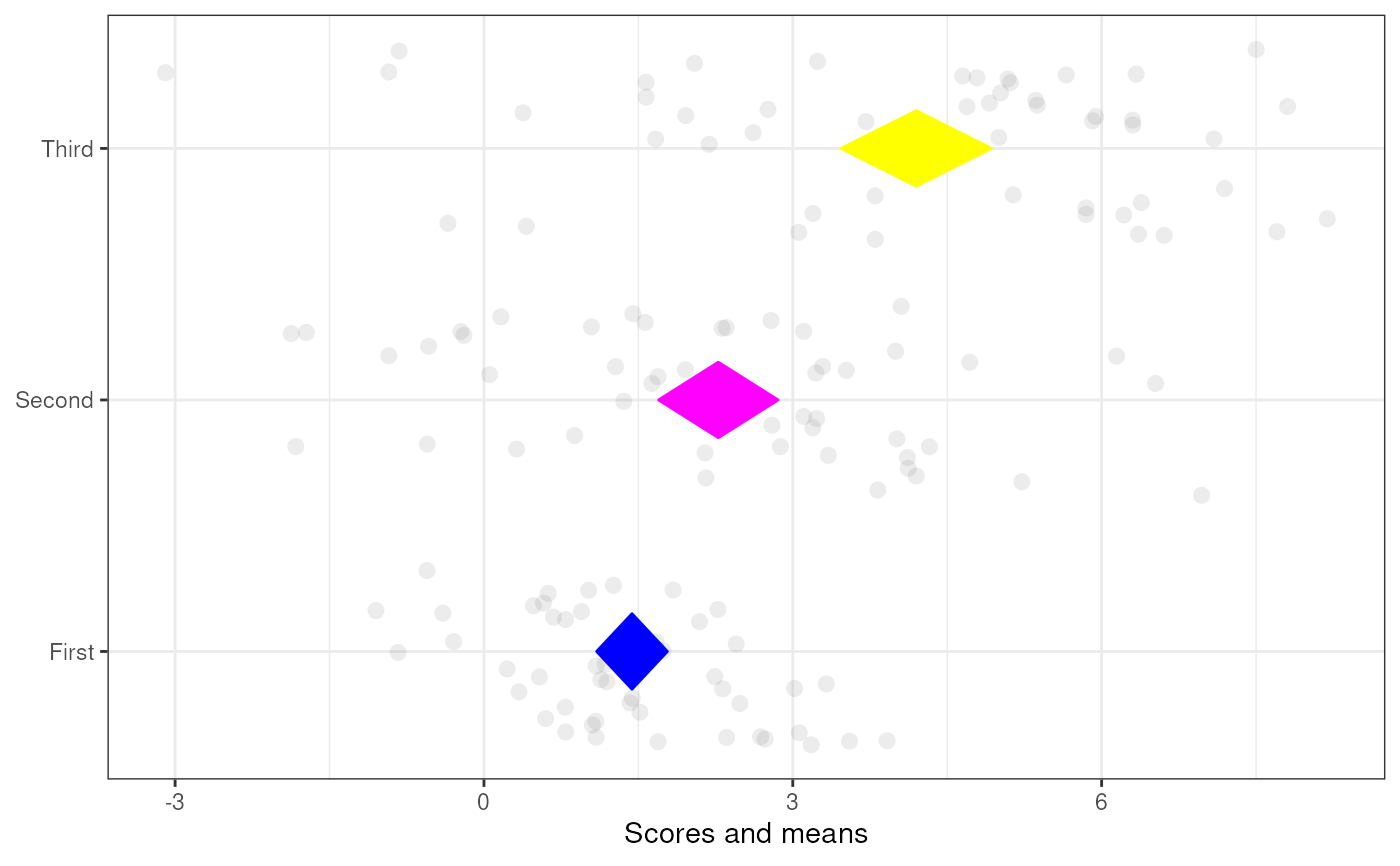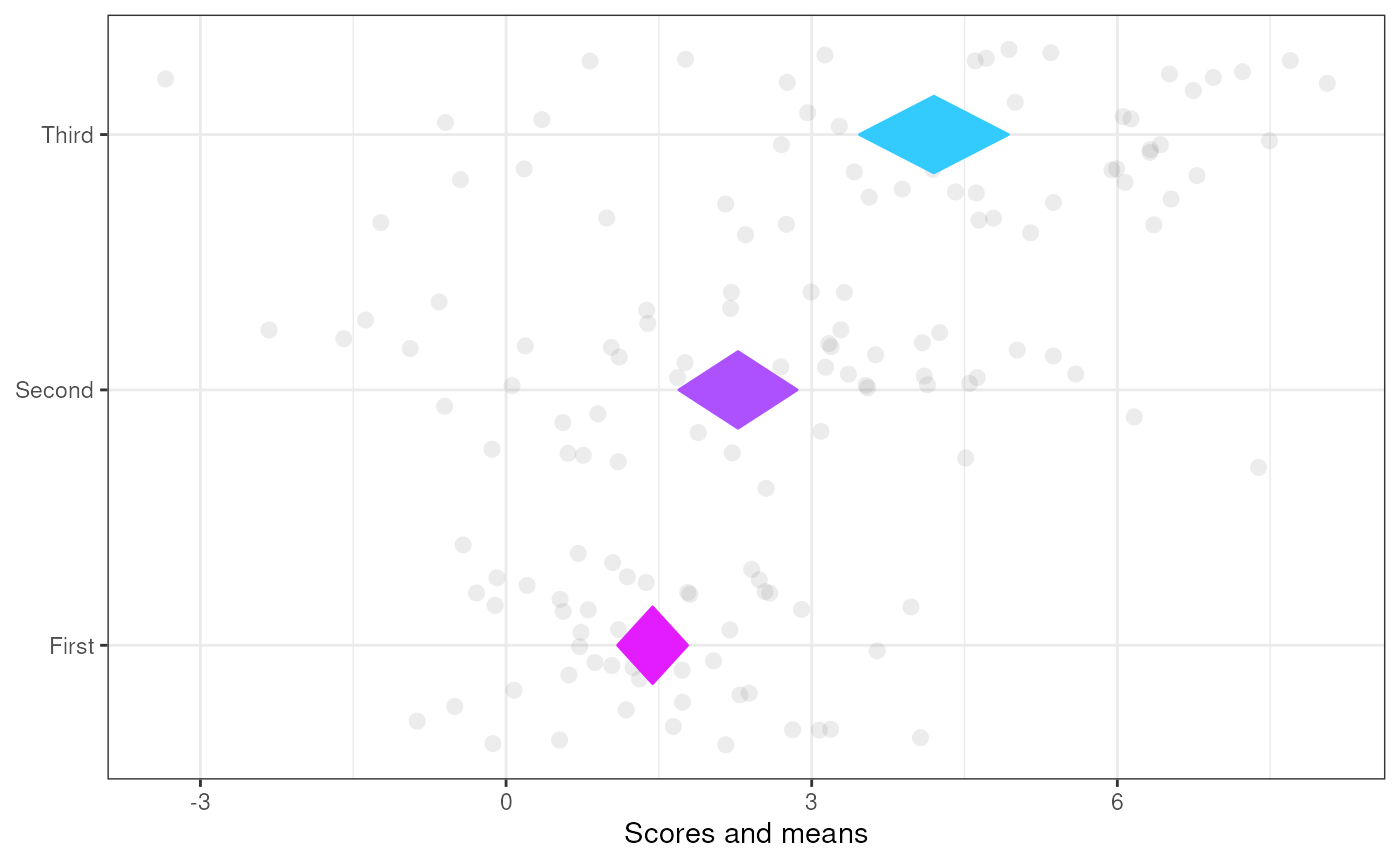This function generates a so-called diamond plot: a plot based on the forest plots that are commonplace in meta-analyses. The underlying idea is that point estimates are uninformative, and it would be better to focus on confidence intervals. The problem of the points with errorbars that are commonly employed is that the focus the audience's attention on the upper and lower bounds, even though those are the least relevant values. Using diamonds remedies this.

meansDiamondPlot(
data,
items = NULL,
labels = NULL,
decreasing = NULL,
conf.level = 0.95,
showData = TRUE,
dataAlpha = 0.1,
dataSize = 3,
dataColor = "#444444",
diamondColors = NULL,
jitterWidth = 0.5,
jitterHeight = 0.4,
returnLayerOnly = FALSE,
xlab = "Scores and means",
ylab = NULL,
theme = ggplot2::theme_bw(),
xbreaks = "auto",
outputFile = NULL,
outputWidth = 10,
outputHeight = 10,
ggsaveParams = ufs::opts\$get("ggsaveParams"),
dat = NULL,
...
)

## Arguments

data, dat

The dataframe containing the variables (items) to show in the diamond plot (the name dat for this argument is deprecated but still works for backward compatibility).

items

Optionally, the names (or numeric indices) of the variables (items) to show in the diamond plot. If NULL, all columns (variables, items) will be used.

labels

A character vector of labels to use instead of column names from the dataframe.

decreasing

Whether to sort the variables (rows) in the diamond plot decreasing (TRUE), increasing (FALSE), or not at all (NULL).

conf.level

The confidence of the confidence intervals.

showData

Whether to show the raw data or not.

dataAlpha

This determines the alpha (transparency) of the data points. Note that argument alpha can be used to set the alpha of the diamonds; this is eventually passed on to ggDiamondLayer().

dataSize

The size of the data points.

dataColor

The color of the data points.

diamondColors

A vector of the same length as there are rows in the dataframe, to manually specify colors for the diamonds.

jitterWidth

How much to jitter the individual datapoints horizontally.

jitterHeight

How much to jitter the individual datapoints vertically.

returnLayerOnly

Set this to TRUE to only return the ggplot() layer of the diamondplot, which can be useful to include it in other plots.

xlab, ylab

The labels of the X and Y axes.

theme

The theme to use.

xbreaks

Where the breaks (major grid lines, ticks, and labels) on the x axis should be.

outputFile

A file to which to save the plot.

outputWidth, outputHeight

Width and height of saved plot (specified in centimeters by default, see ggsaveParams).

ggsaveParams

Parameters to pass to ggsave when saving the plot.

...

Additional arguments are passed to diamondPlot() and eventually to ggDiamondLayer(). This can be used to, for example, specify two or more colors to use to generate a gradient (using generateColors and maybe fullColorRange).

## Value

A ggplot() plot with a ggDiamondLayer() is returned.

See diamondPlot(), meanSDtoDiamondPlot(), ggDiamondLayer(), factorLoadingDiamondCIplot()

## Author

Gjalt-Jorn Peters

Maintainer: Gjalt-Jorn Peters gjalt-jorn@userfriendlyscience.com

## Examples


tmpDf <- data.frame(item1 = rnorm(50, 1.6, 1),
item2 = rnorm(50, 2.6, 2),
item3 = rnorm(50, 4.1, 3));

### A simple diamond plot
meansDiamondPlot(tmpDf);### A diamond plot with manually
### specified labels and colors
meansDiamondPlot(tmpDf,
labels=c('First',
'Second',
'Third'),
diamondColors=c('blue', 'magenta', 'yellow'));### Using a gradient for the colors
meansDiamondPlot(tmpDf,
labels=c('First',
'Second',
'Third'),
generateColors = c("magenta", "cyan"),
fullColorRange = c(1,5));